## Genome-Wide Association Studies

Home
Introduction and Lectures
Intro to the Workshop and Core
Schedule
Dr. Anthony Musolf Talk
What is Bioinformatics/Genomics?
Support
Slack
Zoom
Cheat Sheets
Scripts
Prerequisites
Logging In
CLI
R
Cluster Computing
Data Reduction
Files and Filetypes
Project setup
Preprocessing raw data
Alignment with BWA
Variant calling using GATK
Comparison of freebayes, GATK, and deepvariant output
Data Analysis
Plink Step by Step TDT (solutions)
wAnnovar Annotation
Plink Step by Step (Non FBAT excercise)
Setup in R
GWAS Visualization
ETC
Closing thoughts
Workshop Photos
Github page
Biocore website

# Topics covered in this introduction to R

1. Basic concepts
2. Basic data types in R
3. Import and export data in R
4. Functions in R
5. Basic statistics in R
6. Simple data visulization in R
7. Install packages in R
8. Save data in R session

# Topic 1. Basic concepts

There are three concepts that we should be familiar with before working in R:

• Operators
Assignment Operators in R
Operator Description
<-, = Assignment
Arithmetic Operators in R
Operator Description
\- Subtraction
\* Multiplication
/ Division
^ Exponent
%% Modulus
%/% Integer Division
Relational Operators in R
Operator Description
< Less than
> Greater than
<= Less than or equal to
>= Greater than or equal to
== Equal to
!= Not equal to
Logical Operators in R
Operator Description
! Logical NOT
& Element-wise logical AND
&& Logical AND
| Element-wise logical OR
|| Logical OR
• Functions

Functions are essential in all programming languages. A function takes zero or more parameters and return a result. The way to use a function in R is:

function.name(parameter1=value1, …)

• Variables

A variable is a named storage. The name of a variable can have letters, numbers, dot and underscore. However, a valid variable name cannot start with a underscore or a number, or start with a dot that is followed by a number.

# Simple variables: variables that have a numeric value, a character value (such as a string), or a logical value (True or False)

Examples of numeric values.

``````# assign number 150 to variable a.
a <- 150
a
``````
 150
``````# assign a number in scientific format to variable b.
b <- 3e-2
b
``````
 0.03

Examples of character values.

``````# assign a string "Professor" to variable title
title <- "Professor"
title
``````
 "Professor"
``````# assign a string "Hello World" to variable hello
hello <- "Hello World"
hello
``````
 "Hello World"

Examples of logical values.

``````# assign logical value "TRUE" to variable is_female
is_female <- TRUE
is_female
``````
 TRUE
``````# assign logical value "FALSE" to variable is_male
is_male <- FALSE
is_male
``````
 FALSE
``````# assign logical value to a variable by logical operation
age <- 20
``````
 TRUE

To find out the type of variable.

``````class(is_female)
``````
 "logical"
``````# To check whether the variable is a specific type
is.numeric(hello)
``````
 FALSE
``````is.numeric(a)
``````
 TRUE
``````is.character(hello)
``````
 TRUE

The rule to convert a logical variable to numeric: TRUE > 1, FALSE > 0

``````as.numeric(is_female)
``````
 1
``````as.numeric(is_male)
``````
 0

R does not know how to convert a numeric variable to a character variable.

``````b
``````
 0.03
``````as.character(b)
``````
 "0.03"

# Vectors: a vector is a combination of multiple values(numeric, character or logical) in the same object. A vector is created using the function c() (for concatenate).

``````friend_ages <- c(21, 27, 26, 32)
friend_ages
``````
 21 27 26 32
``````friend_names <- c("Mina", "Ella", "Anna", "Cora")
friend_names
``````
 "Mina" "Ella" "Anna" "Cora"

One can give names to the elements of a vector.

``````# assign names to a vector by specifying them
names(friend_ages) <- c("Mina", "Ella", "Anna", "Carla")
friend_ages
``````
Mina Ella Anna Carla 21 27 26 32
``````# assign names to a vector using another vector
names(friend_ages) <- friend_names
friend_ages
``````
Mina Ella Anna Cora 21 27 26 32

Or One may create a vector with named elements from scratch.

``````friend_ages <- c(Mina=21, Ella=27, Anna=26, Cora=32)
friend_ages
``````
Mina Ella Anna Cora 21 27 26 32

To find out the length of a vector:

``````length(friend_ages)
``````
 4

To access elements of a vector: by index, or by name if it is a named vector.

``````friend_ages
``````
Ella 27
``````friend_ages["Ella"]
``````
Ella 27
``````friend_ages[c(1,3)]
``````
Mina Anna 21 26
``````friend_ages[c("Mina", "Anna")]
``````
Mina Anna 21 26
``````# selecting elements of a vector by excluding some of them.
friend_ages[-3]
``````
Mina Ella Cora 21 27 32

To select a subset of a vector can be done by logical vector.

``````my_friends <- c("Mina", "Ella", "Anna", "Cora")
my_friends
``````
 "Mina" "Ella" "Anna" "Cora"
``````has_child <- c("TRUE", "TRUE", "FALSE", "TRUE")
has_child
``````
 "TRUE" "TRUE" "FALSE" "TRUE"
``````my_friends[has_child == "TRUE"]
``````
 "Mina" "Ella" "Cora"

# Matrices: A matrix is like an Excel sheet containing multiple rows and columns. It is used to combine vectors of the same type.

``````col1 <- c(1,3,8,9)
col2 <- c(2,18,27,10)
col3 <- c(8,37,267,19)

my_matrix <- cbind(col1, col2, col3)
my_matrix
``````
col1 col2 col3 [1,] 1 2 8 [2,] 3 18 37 [3,] 8 27 267 [4,] 9 10 19
``````rownames(my_matrix) <- c("row1", "row2", "row3", "row4")
my_matrix
``````
col1 col2 col3 row1 1 2 8 row2 3 18 37 row3 8 27 267 row4 9 10 19
``````t(my_matrix)
``````
row1 row2 row3 row4 col1 1 3 8 9 col2 2 18 27 10 col3 8 37 267 19

To find out the dimension of a matrix:

``````ncol(my_matrix)
``````
 3
``````nrow(my_matrix)
``````
 4
``````dim(my_matrix)
``````
 4 3

Accessing elements of a matrix is done in similar ways to accessing elements of a vector.

``````my_matrix[1,3]
``````
 8
``````my_matrix["row1", "col3"]
``````
 8
``````my_matrix[1,]
``````
col1 col2 col3 1 2 8
``````my_matrix[,3]
``````
row1 row2 row3 row4 8 37 267 19
``````my_matrix[col3 > 20,]
``````
col1 col2 col3 row2 3 18 37 row3 8 27 267

Calculations with matrices.

``````my_matrix * 3
``````
col1 col2 col3 row1 3 6 24 row2 9 54 111 row3 24 81 801 row4 27 30 57
``````log10(my_matrix)
``````
col1 col2 col3 row1 0.0000000 0.301030 0.903090 row2 0.4771213 1.255273 1.568202 row3 0.9030900 1.431364 2.426511 row4 0.9542425 1.000000 1.278754

Total of each row.

``````rowSums(my_matrix)
``````
row1 row2 row3 row4 11 58 302 38

Total of each column.

``````colSums(my_matrix)
``````
col1 col2 col3 21 57 331

It is also possible to use the function apply() to apply any statistical functions to rows/columns of matrices. The advantage of using apply() is that it can take a function created by user.

The simplified format of apply() is as following:

apply(X, MARGIN, FUN)

X: data matrix MARGIN: possible values are 1 (for rows) and 2 (for columns) FUN: the function to apply on rows/columns

To calculate the mean of each row.

``````apply(my_matrix, 1, mean)
``````
row1 row2 row3 row4 3.666667 19.333333 100.666667 12.666667

To calculate the median of each row

``````apply(my_matrix, 1, median)
``````
row1 row2 row3 row4 2 18 27 10

# Factors: a factor represents categorical or groups in data. The function factor() can be used to create a factor variable.

``````friend_groups <- factor(c(1,2,1,2))
friend_groups
``````
 1 2 1 2 Levels: 1 2

In R, categories are called factor levels. The function levels() can be used to access the factor levels.

``````levels(friend_groups)
``````
 "1" "2"

Change the factor levels.

``````levels(friend_groups) <- c("best_friend", "not_best_friend")
friend_groups
``````
 best_friend not_best_friend best_friend not_best_friend Levels: best_friend not_best_friend

Change the order of levels.

``````levels(friend_groups) <- c("not_best_friend", "best_friend")
friend_groups
``````
 not_best_friend best_friend not_best_friend best_friend Levels: not_best_friend best_friend

By default, the order of factor levels is taken in the order of numeric or alphabetic.

``````friend_groups <- factor(c("not_best_friend", "best_friend", "not_best_friend", "best_friend"))
friend_groups
``````
 not_best_friend best_friend not_best_friend best_friend Levels: best_friend not_best_friend

The factor levels can be specified when creating the factor, if the order does not follow the default rule.

``````friend_groups <- factor(c("not_best_friend", "best_friend", "not_best_friend", "best_friend"), levels=c("not_best_friend", "best_friend"))
friend_groups
``````
 not_best_friend best_friend not_best_friend best_friend Levels: not_best_friend best_friend

If you want to know the number of individuals at each levels, there are two functions.

``````summary(friend_groups)
``````
not_best_friend best_friend 2 2
``````table(friend_groups)
``````
friend_groups not_best_friend best_friend 2 2

# Data frames: a data frame is like a matrix but can have columns with different types (numeric, character, logical).

A data frame can be created using the function data.frame().

``````# creating a data frame using previously defined vectors
friends <- data.frame(name=friend_names, age=friend_ages, child=has_child)
friends
``````
name age child Mina Mina 21 TRUE Ella Ella 27 TRUE Anna Anna 26 FALSE Cora Cora 32 TRUE

To check whether a data is a data frame, use the function is.data.frame().

``````is.data.frame(friends)
``````
 TRUE
``````is.data.frame(my_matrix)
``````
 FALSE

One can convert a object to a data frame using the function as.data.frame().

``````class(my_matrix)
``````
 "matrix"
``````my_data <- as.data.frame(my_matrix)
class(my_data)
``````
 "data.frame"

A data frame can be transposed in the similar way as a matrix.

``````my_data
``````
col1 col2 col3 row1 1 2 8 row2 3 18 37 row3 8 27 267 row4 9 10 19
``````t(my_data)
``````
row1 row2 row3 row4 col1 1 3 8 9 col2 2 18 27 10 col3 8 37 267 19

To obtain a subset of a data frame can be done in similar ways as we have discussed: by index, by row/column names, or by logical values.

``````friends["Mina",]
``````
name age child Mina Mina 21 TRUE
``````# The columns of a data frame can be referred to by the names of the columns
friends
``````
name age child Mina Mina 21 TRUE Ella Ella 27 TRUE Anna Anna 26 FALSE Cora Cora 32 TRUE
``````friends\$age
``````
 21 27 26 32
``````friends[friends\$age > 26,]
``````
name age child Ella Ella 27 TRUE Cora Cora 32 TRUE
``````friends[friends\$child == "TRUE",]
``````
name age child Mina Mina 21 TRUE Ella Ella 27 TRUE Cora Cora 32 TRUE

Function subset() can also be used to get a subset of a data frame.

``````# select friends that are older than 26
subset(friends, age > 26)
``````
name age child Ella Ella 27 TRUE Cora Cora 32 TRUE
``````# select the information of the ages of friends
subset(friends, select=age)
``````
age Mina 21 Ella 27 Anna 26 Cora 32

A data frame can be extended.

``````# add a column that has the information on the marrital status of friends
friends\$married <- c("YES", "YES", "NO", "YES")
friends
``````
name age child married Mina Mina 21 TRUE YES Ella Ella 27 TRUE YES Anna Anna 26 FALSE NO Cora Cora 32 TRUE YES

A data frame can also be extended using the functions cbind() and rbind().

``````# add a column that has the information on the salaries of friends
cbind(friends, salary=c(4000, 8000, 2000, 6000))
``````
name age child married salary Mina Mina 21 TRUE YES 4000 Ella Ella 27 TRUE YES 8000 Anna Anna 26 FALSE NO 2000 Cora Cora 32 TRUE YES 6000

# Lists: a list is an ordered collection of objects, which can be any type of R objects (vectors, matrices, data frames).

A list can be created using the function list().

``````my_list <- list(mother="Sophia", father="John", sisters=c("Anna", "Emma"), sister_age=c(5, 10))
my_list
``````
\$mother  "Sophia" \$father  "John" \$sisters  "Anna" "Emma" \$sister_age  5 10
``````# names of elements in the list
names(my_list)
``````
 "mother" "father" "sisters" "sister_age"
``````# number of elements in the list
length(my_list)
``````
 4

To access elements of a list can be done using its name or index.

``````my_list\$mother
``````
 "Sophia"
``````my_list[["mother"]]
``````
 "Sophia"
``````my_list[]
``````
 "Sophia"
``````my_list[]
``````
 "Anna" "Emma"
``````my_list[]
``````
 "Emma"

# Topic 3. Import and export data in R

R base function read.table() is a general funciton that can be used to read a file in table format. The data will be imported as a data frame.

``````# To read a local file. If you have downloaded the raw_counts.txt file to your local machine, you may use the following command to read it in, by providing the full path for the file location. The way to specify the full path is the same as taught in the command line session. Here we assume raw_counts.txt is in our current working directory

# There is a very convenient way to read files from the internet.
``````

Take a look at the beginning part of the data frame.

``````head(data)
``````
C61 C62 C63 C64 C91 C92 C93 C94 I561 I562 I563 I564 I591 AT1G01010 322 346 256 396 372 506 361 342 638 488 440 479 770 AT1G01020 149 87 162 144 189 169 147 108 163 141 119 147 182 AT1G01030 15 32 35 22 24 33 21 35 18 8 54 35 23 AT1G01040 687 469 568 651 885 978 794 862 799 769 725 715 811 AT1G01046 1 1 5 4 5 3 0 2 4 3 1 0 2 AT1G01050 1447 1032 1083 1204 1413 1484 1138 938 1247 1516 984 1044 1374 I592 I593 I594 I861 I862 I863 I864 I891 I892 I893 I894 AT1G01010 430 656 467 143 453 429 206 567 458 520 474 AT1G01020 156 153 177 43 144 114 50 161 195 157 144 AT1G01030 8 16 24 42 17 22 39 26 28 39 30 AT1G01040 567 831 694 345 575 605 404 735 651 725 591 AT1G01046 8 8 1 0 4 0 3 5 7 0 5 AT1G01050 1355 1437 1577 412 1338 1051 621 1434 1552 1248 1186

Depending on the format of the file, several variants of read.table() are available to make reading a file easier.

read.csv2(): variant used in countries that use a comma “,” as decimal point and a semicolon “;” as field separators.

read.delim(): for reading “tab separated value” files (“.txt”). By default, point(“.”) is used as decimal point.

read.delim2(): for reading “tab separated value” files (“.txt”). By default, comma (“,”) is used as decimal point.

``````# We are going to read a file over the internet by providing the url of the file.

# To look at the file:
``````
C61.C62.C63.C64.C91.C92.C93.C94.I561.I562.I563.I564.I591.I592.I593.I594.I861.I862.I863.I864.I891.I892.I893.I894 1 AT1G01010\t322\t346\t256\t396\t372\t506\t361\t342\t638\t488\t440\t479\t770\t430\t656\t467\t143\t453\t429\t206\t567\t458\t520\t474 2 AT1G01020\t149\t87\t162\t144\t189\t169\t147\t108\t163\t141\t119\t147\t182\t156\t153\t177\t43\t144\t114\t50\t161\t195\t157\t144 3 AT1G01030\t15\t32\t35\t22\t24\t33\t21\t35\t18\t8\t54\t35\t23\t8\t16\t24\t42\t17\t22\t39\t26\t28\t39\t30 4 AT1G01040\t687\t469\t568\t651\t885\t978\t794\t862\t799\t769\t725\t715\t811\t567\t831\t694\t345\t575\t605\t404\t735\t651\t725\t591 5 AT1G01046\t1\t1\t5\t4\t5\t3\t0\t2\t4\t3\t1\t0\t2\t8\t8\t1\t0\t4\t0\t3\t5\t7\t0\t5 6 AT1G01050\t1447\t1032\t1083\t1204\t1413\t1484\t1138\t938\t1247\t1516\t984\t1044\t1374\t1355\t1437\t1577\t412\t1338\t1051\t621\t1434\t1552\t1248\t1186

R base function write.table() can be used to export data to a file.

``````# To write to a file called "output.txt" in your current working directory.
write.table(data2[1:20,], file="output.txt", sep="\t", quote=F, row.names=T, col.names=T)
``````

It is also possible to export data to a csv file.

write.csv()

write.csv2()

\newpage

# Invoking a function by its name, followed by the parenthesis and zero or more arguments.

``````# to find out the current working directory
getwd()
``````
 "/Users/mattsettles/projects/src/github.com-ucdavis-bioinformatics-training/2019_August_UCD_mRNAseq_Workshop/intro2R"
``````# to set a different working directory, use setwd
#setwd("/Users/jli/Desktop")

# to list all variables in the environment
ls()
``````
 "a" "age" "b" "col1"  "col2" "col3" "colFmt" "data"  "data2" "friend_ages" "friend_groups" "friend_names"  "friends" "has_child" "hello" "is_adult"  "is_female" "is_male" "my_data" "my_friends"  "my_list" "my_matrix" "title"
``````# to create a vector from 2 to 3, using increment of 0.1
seq(2, 3, by=0.1)
``````
 2.0 2.1 2.2 2.3 2.4 2.5 2.6 2.7 2.8 2.9 3.0
``````# to create a vector with repeated elements
rep(1:3, times=3)
``````
 1 2 3 1 2 3 1 2 3
``````rep(1:3, each=3)
``````
 1 1 1 2 2 2 3 3 3
``````# to get help information on a function in R: ?function.name
?seq
?sort
?rep
``````
One useful function to find out information on a variable: str().
``````str(data2)
``````
'data.frame': 33602 obs. of 1 variable: \$ C61.C62.C63.C64.C91.C92.C93.C94.I561.I562.I563.I564.I591.I592.I593.I594.I861.I862.I863.I864.I891.I892.I893.I894: chr "AT1G01010\t322\t346\t256\t396\t372\t506\t361\t342\t638\t488\t440\t479\t770\t430\t656\t467\t143\t453\t429\t206\t"| __truncated__ "AT1G01020\t149\t87\t162\t144\t189\t169\t147\t108\t163\t141\t119\t147\t182\t156\t153\t177\t43\t144\t114\t50\t161\t195\t157\t144" "AT1G01030\t15\t32\t35\t22\t24\t33\t21\t35\t18\t8\t54\t35\t23\t8\t16\t24\t42\t17\t22\t39\t26\t28\t39\t30" "AT1G01040\t687\t469\t568\t651\t885\t978\t794\t862\t799\t769\t725\t715\t811\t567\t831\t694\t345\t575\t605\t404\t"| __truncated__ ...

# Two special functions: lapply() and sapply()

lapply() is to apply a given function to every element of a vector and obtain a list as results. The difference between lapply() and apply() is that lapply() can be applied on objects like dataframes, lists or vectors. Function apply() only works on an array of dimension 2 or a matrix.

# To check the syntax of using lapply():

``````#?lapply()

data <- as.data.frame(matrix(rnorm(49), ncol=7), stringsAsFactors=F)
dim(data)
``````
 7 7
``````lapply(1:dim(data), function(x){sum(data[x,])})
``````
[]  0.9394493 []  3.498673 []  2.140273 []  2.096878 []  1.541112 []  0.03483846 []  -1.116205
``````apply(data, MARGIN=1, sum)
``````
 0.93944927 3.49867295 2.14027334 2.09687822 1.54111165 0.03483846  -1.11620494
``````lapply(1:dim(data), function(x){log10(sum(data[x,]))})
``````
Warning in FUN(X[[i]], ...): NaNs produced
[]  -0.02712667 []  0.5439033 []  0.3304692 []  0.3215732 []  0.1878341 []  -1.457941 []  NaN

The function sapply() works like function lapply(), but tries to simplify the output to the most elementary data structure that is possible. As a matter of fact, sapply() is a “wrapper” function for lapply(). By default, it returns a vector.

``````# To check the syntax of using sapply():
#?sapply()

sapply(1:dim(data), function(x){log10(sum(data[x,]))})
``````
Warning in FUN(X[[i]], ...): NaNs produced
 -0.02712667 0.54390335 0.33046924 0.32157321 0.18783410 -1.45794110  NaN

If the “simplify” parameter is turned off, sapply() will produced exactly the same results as lapply(), in the form of a list. By default, “simplify” is turned on.

``````sapply(1:dim(data), function(x){log10(sum(data[x,]))}, simplify=FALSE)
``````
Warning in FUN(X[[i]], ...): NaNs produced
[]  -0.02712667 []  0.5439033 []  0.3304692 []  0.3215732 []  0.1878341 []  -1.457941 []  NaN

# Topic 5. Basic statistics in R

Description R_function
Mean mean()
Standard deviation sd()
Variance var()
Minimum min()
Maximum max()
Median median()
Range of values: minimum and maximum range()
Sample quantiles quantile()
Generic function summary()
Interquartile range IQR()

Calculate the mean expression for each sample.

``````apply(data, 2, mean)
``````
V1 V2 V3 V4 V5 V6 0.38447484 0.49181913 -0.35523947 -0.44494529 0.08749222 0.21590775 V7 0.92549352

Calculate the range of expression for each sample.

``````apply(data, 2, range)
``````
V1 V2 V3 V4 V5 V6 [1,] -1.057522 -1.594726 -1.319862 -2.0252357 -0.9448364 -0.9075704 [2,] 2.195689 1.745992 1.236270 0.9487987 1.1573999 1.3134008 V7 [1,] -0.1891506 [2,] 2.2566140

Calculate the quantiles of each samples.

``````apply(data, 2, quantile)
``````
V1 V2 V3 V4 V5 V6 0% -1.0575225 -1.5947263 -1.3198616 -2.0252357 -0.9448364 -0.9075704 25% -0.4041226 0.3554335 -0.8752390 -1.3243273 -0.5317714 -0.6560503 50% -0.2863864 0.8034528 -0.4286163 -0.4850001 -0.0371838 0.3668206 75% 1.3238943 0.8885743 -0.1119954 0.5477373 0.7503043 1.0254019 100% 2.1956895 1.7459918 1.2362704 0.9487987 1.1573999 1.3134008 V7 0% -0.1891506 25% 0.2454125 50% 1.0015498 75% 1.4593082 100% 2.2566140

# Topic 6. Simple data visulization in R

Scatter plot and line plot can be produced using the function plot().

``````x <- c(1:50)
y <- 1 + sqrt(x)/2
plot(x,y)
``````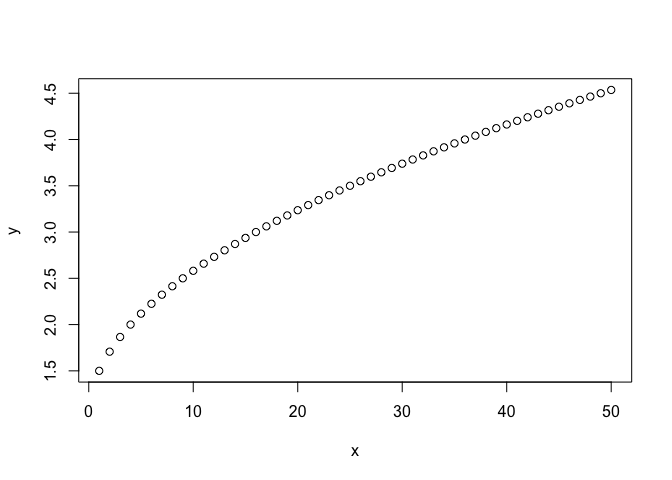``````plot(x,y, type="l")
``````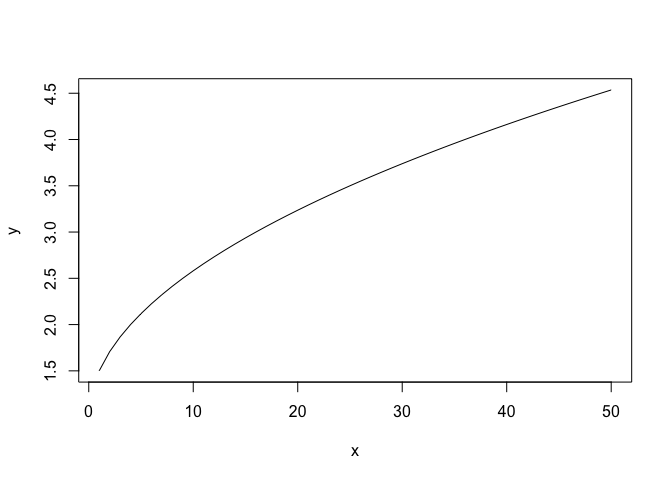``````# plot both the points and lines
first plot points
plot(x,y)
lines(x,y, type="l")
``````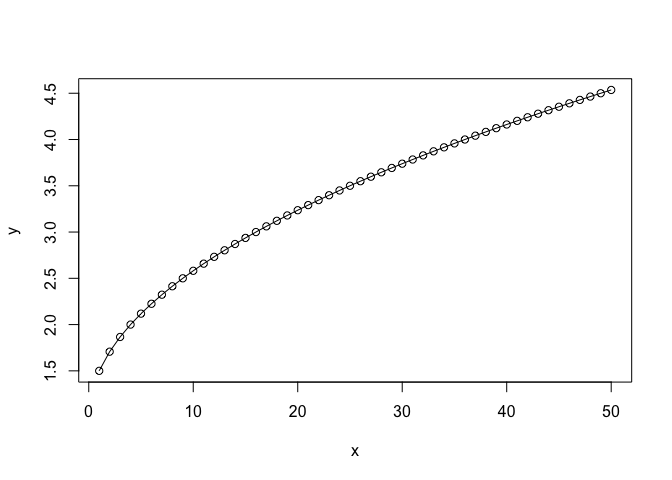`````` lines() can only be used to add information to a graph, while it cannot produce a graph on its own.
``````

boxplot() can be used to summarize data.

``````boxplot(data, xlab="Sample ID", ylab="Raw Counts")
``````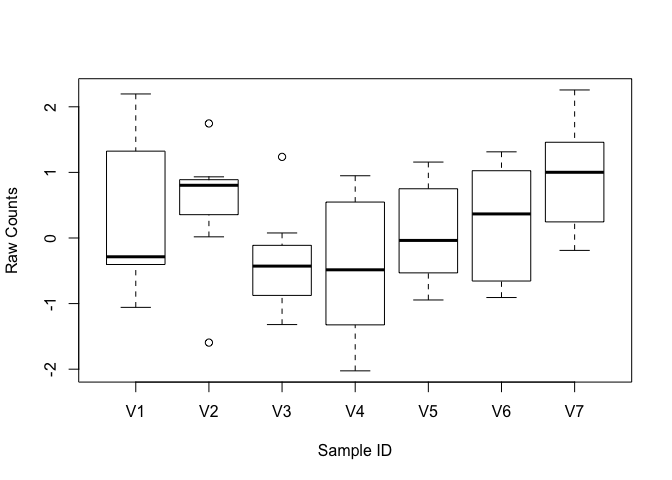add more details to the plot.

``````boxplot(data, xlab="Sample ID", ylab="Raw Counts", main="Expression levels", col="blue", border="black")
``````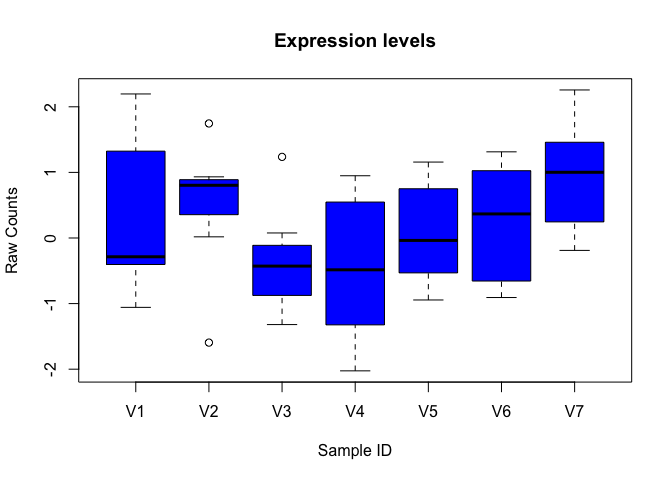``````x <- rnorm(1000)
boxplot(x)
``````hist() can be used to create histograms of data.

``````hist(x)
``````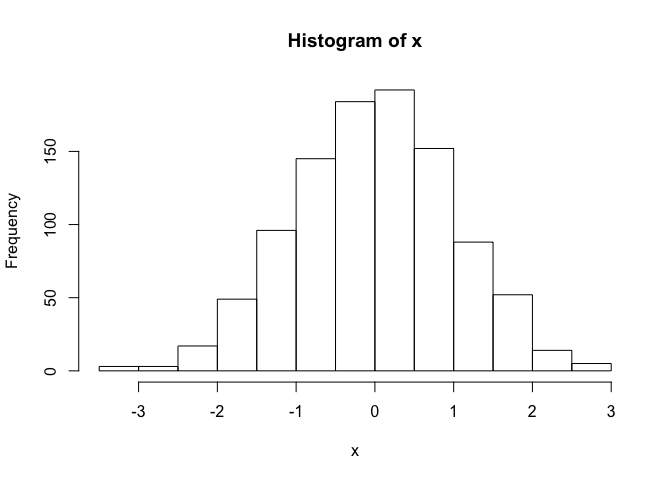``````# use user defined break points
hist(x, breaks=seq(range(x)-1, range(x)+1, by=0.5))
``````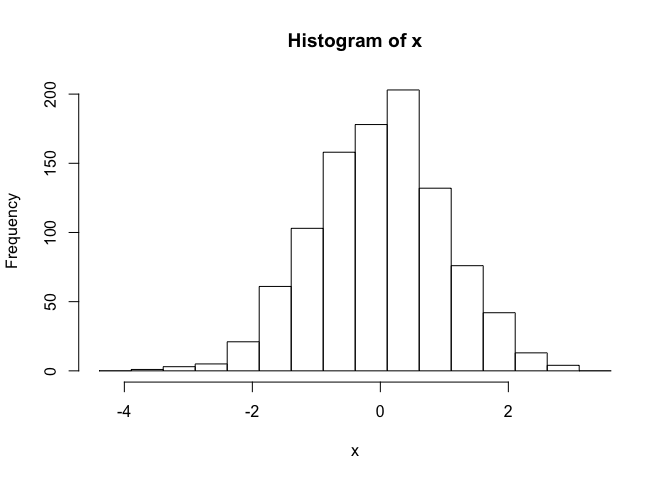``````# clear plotting device/area
dev.off()
``````
null device 1

# Topic 7. Install packages in R

Starting from Bioconductor version 3.8, the installation of packages is recommended to use BiocManager.

``````if (!requireNamespace("BiocManager"))
install.packages("BiocManager")
install core packages
BiocManager::install()
install specific packages
``````
• Bioconductor has a repository and release schedule that differ from R (Bioconductor has a ‘devel’ branch to which new packages and updates are introduced, and a stable ‘release’ branch emitted once every 6 months to which bug fixes but not new features are introduced). This mismatch causes that the version detected by install.packages() is sometimes not the most recent ‘release’.

• A consequence of the distince ‘devel’ branch is that install.packages() sometimes points only to the ‘release’ repository, while users might want to have access to the leading-edge features in the develop version.

• An indirect consequence of Bioconductor’s structured release is that packages generally have more extensive dependences with one another.

# It is always recommended to update to the most current version of R and Bioconductor. If it is not possible and R < 3.5.0, please use the legacy approach to install Bioconductor packages

``````source("http://bioconductor.org/biocLite.R")
install core packages
biocLite()
install specific packages
biocLite("RCircos")
biocLite(c("IdeoViz", "devtools"))
``````

The R function install.packages() can be used to install packages that are not part of Bioconductor.

``````install.packages("ggplot2", repos="http://cran.us.r-project.org")
``````

Install from source of github.

``````library(devtools)
#install_github("stephenturner/qqman")
``````

# Topic 8. Save data in R session

To save history in R session

``````#savehistory(file="March27.history")

``````

To save objects in R session

``````save(list=c("x", "data"), file="March27.RData")